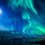# Electrostatics (Doubt)

Consider two conducting spheres of same radius $r$, separated by a distance $d$. Charges $+q$ and $-q$ are placed on the two spheres. Find the force $F$ acting on one sphere due to the other.

###### Problem credit: Ritvik VantipalliNote by Venkata Karthik Bandaru
4 years, 4 months ago

This discussion board is a place to discuss our Daily Challenges and the math and science related to those challenges. Explanations are more than just a solution — they should explain the steps and thinking strategies that you used to obtain the solution. Comments should further the discussion of math and science.

When posting on Brilliant:

• Use the emojis to react to an explanation, whether you're congratulating a job well done , or just really confused .
• Ask specific questions about the challenge or the steps in somebody's explanation. Well-posed questions can add a lot to the discussion, but posting "I don't understand!" doesn't help anyone.
• Try to contribute something new to the discussion, whether it is an extension, generalization or other idea related to the challenge.

MarkdownAppears as
*italics* or _italics_ italics
**bold** or __bold__ bold
- bulleted- list
• bulleted
• list
1. numbered2. list
1. numbered
2. list
Note: you must add a full line of space before and after lists for them to show up correctly
paragraph 1paragraph 2

paragraph 1

paragraph 2

[example link](https://brilliant.org)example link
> This is a quote
This is a quote
    # I indented these lines
# 4 spaces, and now they show
# up as a code block.

print "hello world"
# I indented these lines
# 4 spaces, and now they show
# up as a code block.

print "hello world"
MathAppears as
Remember to wrap math in $$ ... $$ or $ ... $ to ensure proper formatting.
2 \times 3 $2 \times 3$
2^{34} $2^{34}$
a_{i-1} $a_{i-1}$
\frac{2}{3} $\frac{2}{3}$
\sqrt{2} $\sqrt{2}$
\sum_{i=1}^3 $\sum_{i=1}^3$
\sin \theta $\sin \theta$
\boxed{123} $\boxed{123}$

Sort by:

Can you please elaborate the question?

- 4 years, 4 months ago

@Kishore S Shenoy There are two spherical conductors : one having charge +q and other -q. Find force of interaction between them ( as a function of their common radius r and distance between their centres d).

- 4 years, 4 months ago

Ohh, the separation is not large? I suppose that is the catch?

- 4 years, 4 months ago

@Kishore S Shenoy The separation is arbitary (of course, greater than 2r).

- 4 years, 4 months ago

- 4 years, 4 months ago

@Kishore S Sheno Nope, I don't know the answer.

- 4 years, 4 months ago

Consider the charge centred at the centre. Force sud be q^2/(d+2r)^2

I am guessing. Am I right?

- 3 years, 6 months ago

No. The spheres are conducting. Hence, the charges distribute in such a way that the potential at the surface is equal radially symmetric.

- 3 years, 4 months ago